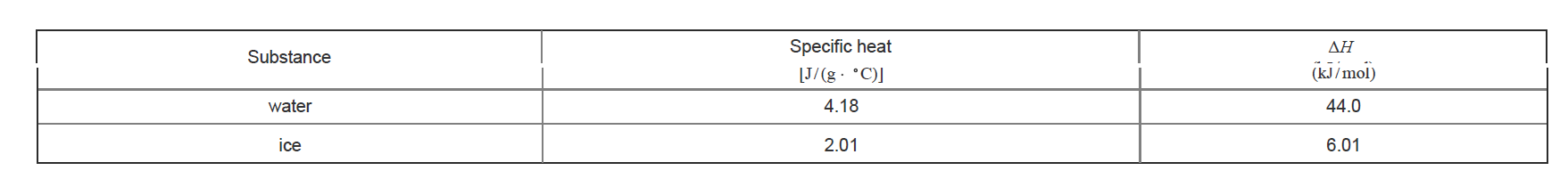# Problem: Calculate the enthalpy change, ΔH, for the process in which 38.9 g of water is converted from liquid at 10.4°C to vapor at 25.0°C . For water, ΔHvap = 44.0 kJ/mol at 25.0°C and Cs = 4.18 J/ (g°C) for H2O(l).Express your answer to three significant figures and include the appropriate units.Heat, q, is energy transferred between a system and its surroundings. For a process that involves a temperature change q = mCsΔTwhere Cs is specific heat and mm is mass.Heat can also be transferred at a constant temperature when there is a change in state. For a process that involves a phase changeq = n ⋅ ΔHwhere, n is the number of moles and ΔH is the enthalpy of fusion, vaporization, or sublimation.The following table provides the specific heat and enthalpy changes for water and ice.

###### FREE Expert Solution
100% (108 ratings)View Complete Written Solution
###### Problem Details

Calculate the enthalpy change, ΔH, for the process in which 38.9 g of water is converted from liquid at 10.4°C to vapor at 25.0°C . For water, ΔHvap = 44.0 kJ/mol at 25.0°C and Cs = 4.18 J/ (g°C) for H2O(l).

Express your answer to three significant figures and include the appropriate units.

Heat, q, is energy transferred between a system and its surroundings. For a process that involves a temperature change

q = mCsΔT
where Cs is specific heat and mm is mass.

Heat can also be transferred at a constant temperature when there is a change in state. For a process that involves a phase change

q = n ⋅ ΔH

where, n is the number of moles and ΔH is the enthalpy of fusion, vaporization, or sublimation.

The following table provides the specific heat and enthalpy changes for water and ice.